# BPSK Bit Error Rate Calculation Using Python

Have you ever thought about how life would be without MATLAB. As it turns out there are free and open source options such as Python. We have so far restricted ourselves to MATLAB in this blog but now we venture out to find out what are the other options. Given below is a most basic Python code that calculates the Bit Error Rate of Binary Phase Shift Keying (BPSK). Compare this to our MATLAB implementation earlier [BPSK BER].

There are various IDEs available for writing your code but I have used Enthought Canopy Editor (32 bit) which is free to download and is also quite easy to use [download here]. So as it turns out that there is life beyond MATLAB. In fact there are several advantages of using Python over MATLAB which we will discuss later in another post. Lastly please note the indentation in the code below as there is no “end” statement in a “for loop” in Python.

```from numpy import sqrt
from numpy.random import rand, randn
import matplotlib.pyplot as plt

N = 5000000
EbNodB_range = range(0,11)
itr = len(EbNodB_range)
ber = [None]*itr

for n in range (0, itr):

EbNodB = EbNodB_range[n]
EbNo=10.0**(EbNodB/10.0)
x = 2 * (rand(N) >= 0.5) - 1
noise_std = 1/sqrt(2*EbNo)
y = x + noise_std * randn(N)
y_d = 2 * (y >= 0) - 1
errors = (x != y_d).sum()
ber[n] = 1.0 * errors / N

print "EbNodB:", EbNodB
print "Error bits:", errors
print "Error probability:", ber[n]

plt.plot(EbNodB_range, ber, 'bo', EbNodB_range, ber, 'k')
plt.axis([0, 10, 1e-6, 0.1])
plt.xscale('linear')
plt.yscale('log')
plt.xlabel('EbNo(dB)')
plt.ylabel('BER')
plt.grid(True)
plt.title('BPSK Modulation')
plt.show()
```

MATLAB vs PYTHON A COMPARISON

# Theoretical BER of M-QAM in Rayleigh Fading

We have previously discussed the Bit Error Rate of M-QAM in Rayleigh Fading using Monte Carlo Simulation. We now turn our attention to calculation of Bit Error Rate (BER) of M-QAM in Rayleigh fading using analytical techniques. In particular we look at the method used in MATLAB function berfading.m. In this function the BER of 4-QAM, 16-QAM and 64-QAM is calculated from series expressions having 1, 3 and 5 terms respectively. These are given below (M is the constellation size and must be a power of 2).

```if (M == 4)
ber = 1/2 * ( 1 - sqrt(gamma_c/k./(1+gamma_c/k)) );
elseif (M == 16)
ber = 3/8 * ( 1 - sqrt(2/5*gamma_c/k./(1+2/5*gamma_c/k)) ) ...
+ 1/4 * ( 1 - sqrt(18/5*gamma_c/k./(1+18/5*gamma_c/k)) ) ...
- 1/8 * ( 1 - sqrt(10*gamma_c/k./(1+10*gamma_c/k)) );
elseif (M == 64)
ber = 7/24 * ( 1 - sqrt(1/7*gamma_c/k./(1+1/7*gamma_c/k)) ) ...
+ 1/4 * ( 1 - sqrt(9/7*gamma_c/k./(1+9/7*gamma_c/k)) ) ...
- 1/24 * ( 1 - sqrt(25/7*gamma_c/k./(1+25/7*gamma_c/k)) ) ...
+ 1/24 * ( 1 - sqrt(81/7*gamma_c/k./(1+81/7*gamma_c/k)) ) ...
- 1/24 * ( 1 - sqrt(169/7*gamma_c/k./(1+169/7*gamma_c/k)) );
```

Although using these expressions we get very accurate BER but it is not that simple to calculate (the expressions become even more complicated for higher constellation sizes such as 256-QAM). Therefore we try to simplify these expressions by using only the first term in each expression. To our surprise the results match quite well with the results using the exact formulae. There is very minor difference at low signal to noise ratios but that can be easily bargained for the ease of calculation.

So here is our program for calculating the BER using the approximate method.

```%%%%%%%%%%%%%%%%%%%%%%%%%%%%%%%%%%%%%%%%%%%%%%%%%%%%%%%%%%%%%%%%%%%%%%%%%%%
% FUNCTION TO CALCULATE THE BER OF M-QAM IN RAYLEIGH FADING
% M: Input, Constellation Size
% EbNo: Input, Energy Per Bit to Noise Power Spectral Density
% ber: Output, Bit Error Rate
%%%%%%%%%%%%%%%%%%%%%%%%%%%%%%%%%%%%%%%%%%%%%%%%%%%%%%%%%%%%%%%%%%%%%%%%%%%

k=log2(M);
EbNoLin=10.^(EbNo/10);
gamma_c=EbNoLin*k;

if M==4
%4-QAM
ber = 1/2 * ( 1 - sqrt(gamma_c/k./(1+gamma_c/k)) );
elseif M==16
%16-QAM
ber = 3/8 * ( 1 - sqrt(2/5*gamma_c/k./(1+2/5*gamma_c/k)) );
elseif M==64
%64-QAM
ber = 7/24 * ( 1 - sqrt(1/7*gamma_c/k./(1+1/7*gamma_c/k)) );
else
%Warning
warning('M=4,16,64')
ber=zeros(1,length(EbNo));
end

semilogy(EbNo,ber,'o-')
xlabel('EbNo(dB)')
ylabel('BER')
axis([0 24 0.001 1])
grid on

return
%%%%%%%%%%%%%%%%%%%%%%%%%%%%%%%%%%%%%%%%%%%%%%%%%%%%%%%%%%%%%%%%%%%%%%%%%%%
```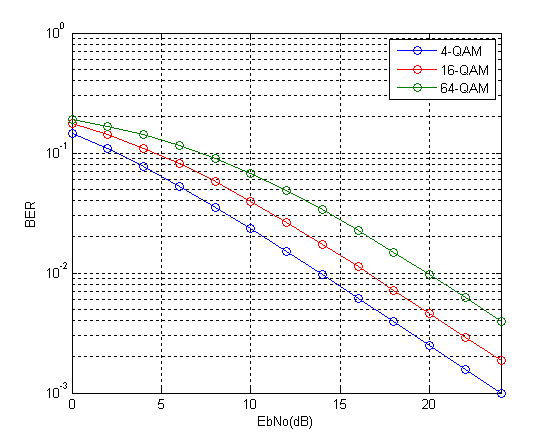So we see that the results match quite well with the results previously obtained through simulation. We will next tackle the problem of simplifying the expression for higher order modulations such as 256-QAM in both Rayleigh and Ricean channels.

# BER of 64-QAM OFDM in Frequency Selective Fading-II

In the previous post we had considered a static frequency-selective channel. We now consider a time-varying frequency selective channel with 7 taps. Each tap of the time domain filter has a Gaussian distributed real component with variance 1/(2*n_tap) and a Gaussian distributed imaginary component with variance 1/(2*n_tap). The amplitude of each tap is thus Rayleigh distributed and the phase is Uniformly distributed. Since the power in each component is normalized by the filter length (n_tap) the BER performance would remain the same even if the filter length is changed (this has been verified experimentally).

```%%%%%%%%%%%%%%%%%%%%%%%%%%%%%%%%%%%%%%%%%%%%%%%%%%%%%%%%%%%%%%%%%%%%%%%%%
% FUNCTION TO SIMULATE PERFORMANCE OF 64-OFDM IN TIME VARYING FREQUENCY SELECTIVE CHANNEL
% n_bits: Input, length of binary sequence
% n_fft: Input, length of FFT (Fast Fourier Transform)
% EbNodB: Input, energy per bit to noise power spectral density ratio
% ber: Output, bit error rate
%%%%%%%%%%%%%%%%%%%%%%%%%%%%%%%%%%%%%%%%%%%%%%%%%%%%%%%%%%%%%%%%%%%%%%%%%

Eb=7;
M=64;
k=log2(M);
n_cyc=32;
EbNo=10^(EbNodB/10);
x=transpose(round(rand(1,n_bits)));
h1=modem.qammod(M);
h1.inputtype='bit';
h1.symbolorder='gray';
y=modulate(h1,x);
n_sym=length(y)/n_fft;
n_tap=7;

for n=1:n_sym;
s_ofdm=sqrt(n_fft)*ifft(y((n-1)*n_fft+1:n*n_fft),n_fft);
s_ofdm_cyc=[s_ofdm(n_fft-n_cyc+1:n_fft); s_ofdm];
ht=(1/sqrt(2))*(1/sqrt(n_tap))*(randn(1,n_tap)+j*randn(1,n_tap));
Hf=fft(ht,n_fft);
r_ofdm_cyc=conv(s_ofdm_cyc,ht);
r_ofdm_cyc=(r_ofdm_cyc(1:n_fft+n_cyc));
wn=sqrt((n_fft+n_cyc)/n_fft)*(randn(1,n_fft+n_cyc)+j*randn(1,n_fft+n_cyc));
r_ofdm_cyc=r_ofdm_cyc+sqrt(Eb/(2*EbNo))*wn.';
r_ofdm=r_ofdm_cyc(n_cyc+1:n_fft+n_cyc);
s_est((n-1)*n_fft+1:n*n_fft)=(fft(r_ofdm,n_fft)/sqrt(n_fft))./Hf.';
end

h2=modem.qamdemod(M);
h2.outputtype='bit';
h2.symbolorder='gray';
h2.decisiontype='hard decision';
z=demodulate(h2,s_est.');
ber=(n_bits-sum(x==z))/n_bits
return
%%%%%%%%%%%%%%%%%%%%%%%%%%%%%%%%%%%%%%%%%%%%%%%%%%%%%%%%%%%%%%%%%%%%%%%%%
```

As before we have used an FFT size of 128 and cyclic prefix of 32 samples. The FFT and IIFT operations are normalized to maintain the signal to noise ratio (SNR). The extra energy transmitted in the cyclic prefix is also accounted for in the SNR calibration.

It is observed that the BER performance of 64-QAM OFDM in the time-varying frequency-selective channel is quite similar to that in the static frequency-selective channel with complex filter taps. It must be noted that with 64-QAM the goal is to achieve higher bit rate, error rates can be improved using antenna diversity and channel coding schemes.

Given below is the wrapper that should be used along with the above code. The wrapper basically calls the above routine for each value of EbNodB. The length of the binary sequence and the FFT size are other inputs to the function. The bit error rate at the specific EbNodB is the output of the function.

```%%%%%%%%%%%%%%%%%%%%%%%%%%%%%%%%%%%
clear all;
close all;
k=6;
n_fft=128;
l=k*n_fft*1e3;
EbNodB=0:2:20;
for n=1:length(EbNodB);n
end;
semilogy(EbNodB,ber,'O-');
grid on
xlabel('EbNo')
ylabel('BER')
%%%%%%%%%%%%%%%%%%%%%%%%%%%%%%%%%%%```

In future we would use the standard LTE channel models, namely EPA, EVA and ETU in our simulation.

# Bit Error Rate of QPSK

Simulating a QPSK system is equivalent to simulating two BPSK systems in parallel. So there is no difference in bit error rate(BER). Since the simulation is at baseband we multiply the in-phase and quadrature streams by 1 and j respectively (instead of cos and sin carriers). At the receiver we just use the real and imag functions to separate the two symbol streams. The BER is the average BER of the two parallel streams.

As in the case of BPSK we can show that the baseband representation (using 1 and j)  is equivalent to using the passband representation (using cosine and sine). Lets assume the following signal model for QPSK.

s(t)=a(t)*cos(2*pi*f*t)+b(t)*sin(2*pi*f*t)

where a(t) and b(t) contain the information to be transmitted over the channel. Now at the receiver we multiply this signal with cos( ) to recover a(t) and sin( ) to recover b(t).

cos(2*pi*f*t)*s(t)

=cos(2*pi*f*t) [a(t)*cos(2*pi*f*t)+b(t)*sin(2*pi*f*t)]

=a(t)*cos(2*pi*f*t)*cos(2*pi*f*t) +b(t)*sin(2*pi*f*t)*cos(2*pi*f*t)

=(a(t)/2)*(1+cos(4*pi*f*t))+(b(t)/2)*sin(4*pi*f*t)

=(a(t)/2)+(a(t)/2)*cos(4*pi*f*t)+(b(t)/2)*sin(4*pi*f*t)

After low pass filtering (LPF) we get (a(t)/2), which it the required in-phase component scaled by a constant 1/2. Similarly we can find the quadrature component b(t).

sin(2*pi*f*t)*s(t)

=sin(2*pi*f*t) [a(t)*cos(2*pi*f*t)+b(t)*sin(2*pi*f*t)]

=a(t)*sin(2*pi*f*t)*cos(2*pi*f*t)+b(t)*sin(2*pi*f*t)*sin(2*pi*f*t)

=(a(t)/2)*sin(4*pi*f*t)+(b(t)/2)*(1-cos(4*pi*f*t))

=(a(t)/2)*sin(4*pi*f*t)+(b(t)/2)-(b(t)/2)cos(4*pi*f*t)

Again after low pass filtering (LPF) we are left with the quadrature component b(t) scaled by the constant 1/2. So we can conclude that the multiplication by the carrier terms at the transmitter and receiver is not required in simulation and we can simply transmit a(t) and b(t). We just have to make sure that a(t) and b(t) are orthogonal to each other so that they do not interfere.

So the transmitted QPSK signal would have the form.

s(t)=a(t)+j*b(t)

The steps involved in the simulation are

1. Generate a random sequence of symbols for the in-phase and quadrature components (-1 corresponding to binary value of 0 and +1 corresponding to binary value of 1). Add the in-phase and quadrature components in the form a(t)+j*b(t).

2. Generate complex samples of Additive White Gaussian Noise (AWGN) with the required variance (noise power = noise variance OR noise power = square of noise standard deviation OR noise power = noise power spectral density * signal bandwidth).

3. Add AWGN samples to the QPSK signal.

4. Detection is performed at the receiver by determining the sign of the in-phase and quadrature components.

5. And finally the bit error rate (BER) is calculated for the in-phase and quadrature components. Total bit error rate is the mean of the two values.

```%%%%%%%%%%%%%%%%%%%%%%%%%%%%%%%%%%%%%%%%%%%%%%%%%%%%%%%%%%%%%%%%%%%%%%%%%%%%
% FUNCTION TO CALCULATE BER OF QPSK IN AWGN
% l - Input, length of the symbol sequence
% EbNo - Input, energy per bit to noise power spectral density
% ber - Output, bit error rate
%%%%%%%%%%%%%%%%%%%%%%%%%%%%%%%%%%%%%%%%%%%%%%%%%%%%%%%%%%%%%%%%%%%%%%%%%%%%
function[ber]=err_rate2(l,EbNo)
si=2*(round(rand(1,l))-0.5);               % In-phase component
s=si+j*sq;                              % QPSK symbol
n=(1/sqrt(2*10^(EbNo/10)))*(randn(1,l)+j*randn(1,l)); % AWGN Noise
r=s+n;                                  % Received signal with noise
si_=sign(real(r));                        % Detected in-phase component
ber1=(l-sum(si==si_))/l;                  % Bit error rate in-phase
ber2=(l-sum(sq==sq_))/l;                  % Bit error rate quadrature
ber=mean([ber1 ber2]);                    % Mean bit error rate
return
%%%%%%%%%%%%%%%%%%%%%%%%%%%%%%%%%%%%%%%%%%%%%%%%%%%%%%%%%%%%%%%%%%%%%%%%%%%%
```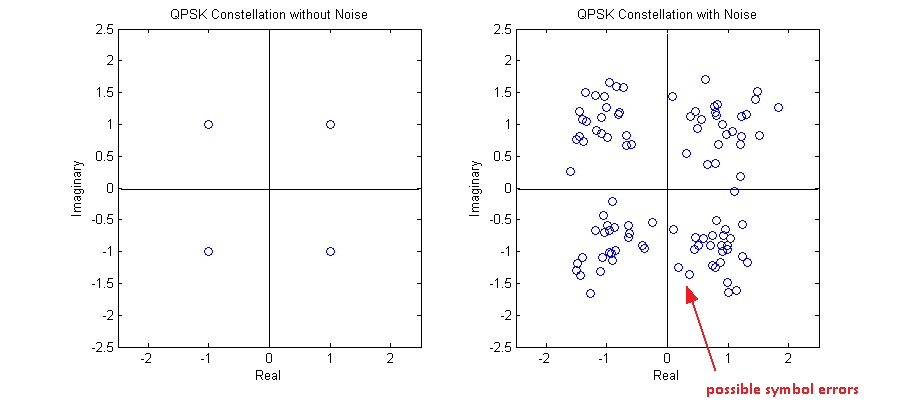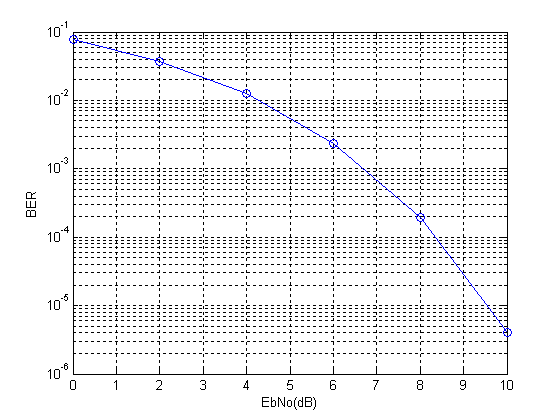One final comment that I want to make is that bit error rate and symbol error rate is not always the same. Taking the example of QPSK a symbol error might occur when there is an error in the in-phase stream or the quadrature stream or both. So it is not a one to one mapping!!!

Note:

1. For a QPSK constellation centered around the origin of the co-ordinate system, the decision boundaries are simply defined by the x and y axes.

2. The reason for the name QPSK is that there are four symbols in the constellation, each having one of four possible phases (45, 135, 225, 315).

# Bit Error Rate of BPSK

Modulation is the process by which a binary stream (zeros and ones) is converted to a format that is suitable for transmission over a wired or wireless channel that is prone to noise and interference as well as distortion. The most basic modulation scheme is BPSK or Binary Phase Shift Keying. It transmits the information in the phase of the signal which could be one of two values (0 degrees or 180 degrees).

BPSK signal can be represented as (called the passband representation)

s(t)=a(t)*cos(2*pi*f*t)

where a(t) is a time varying parameter which can have one of two values (+1 or -1). This is equivalent to having the phase of the carrier rotated by 0 degrees or 180 degrees. In simulation of digital communications systems we usually take out the carrier and perform the simulation at baseband. The passband and baseband simulations are equivalent because the carrier signal introduced at the transmitter can be easily removed at the receiver by a process called correlation (or simply put multiplication by the carrier followed by low pass filtering) and what we are left with is the parameter a(t).

If the transmitted signal is given as

a(t)*cos(2*pi*f*t)

then by multiplication with the carrier at the receiver we get

a(t)*cos(2*pi*f*t)*cos(2*pi*f*t)

=(a(t)/2)*(1+cos(4*pi*f*t))

and after low pass filtering the cosine term at twice the carrier frequency is removed and we get the parameter a(t) scaled by the factor 1/2. Since the information is contained in the sign of the parameter a(t) we can recover our transmitted symbols.

So in simulation, instead of multiplying the parameter a(t) by the carrier at transmitter and then again at the receiver we simply transmit a(t). This is equivalent to simulation of a Pulse Amplitude Modulation (PAM) system with two levels. Following are the steps involved in the simulation of BPSK system.

Steps:

1. Generate a random sequence of symbols (+1,-1)

2. Generate samples of Additive White Gaussian Noise (AWGN) with the required variance (noise power = noise variance OR noise power = square of noise standard deviation OR noise power = noise power spectral density * signal bandwidth).

3. Add AWGN samples to the BPSK signal.

4. Detection is performed at the receiver by determining the sign of the parameter a(t).

5. And finally the bit error rate (BER) is calculated. Which is the same as symbol error rate (SER) in this case.

Given below is the MATLAB code that performs these functions. Also shown below are the signals generated at the first four steps and the bit error rate calculated in the fifth and last step.

```%%%%%%%%%%%%%%%%%%%%%%%%%%%%%%%%%%%%%%%%%%%%%%%%%%%%%%%%%%%%%%%%%%%%%
% FUNCTION TO CALCULATE BER OF BPSK IN AWGN
% l - Input, length of the symbol sequence
% EbNo - Input, energy per bit to noise power spectral density in dB
% ber - Output, bit error rate
%%%%%%%%%%%%%%%%%%%%%%%%%%%%%%%%%%%%%%%%%%%%%%%%%%%%%%%%%%%%%%%%%%%%%
function[ber]=err_rate(l,EbNo)
s=2*(round(rand(1,l))-0.5);             % Generate BPSK symbols
n=(1/sqrt(2*10^(EbNo/10)))*randn(1,l);  % Generate AWGN noise
r=s+n;                                  % Add noise to signal
s_=sign(r);                             % Detect symbols
ber=(l-sum(s==s_))/l;                   % Calculate BER
return
%%%%%%%%%%%%%%%%%%%%%%%%%%%%%%%%%%%%%%%%%%%%%%%%%%%%%%%%%%%%%%%%%%%%%
```

The length of the symbol sequence and EbNo (a bit different than SNR) are the inputs to the function and the bit error rate (BER) is the output. The length of the sequence must be such that you can count about 25 symbol errors at each value of EbNo. This means that at an EbNo of 10dB you would need to pass a few million symbols through the channel. Try it out!!!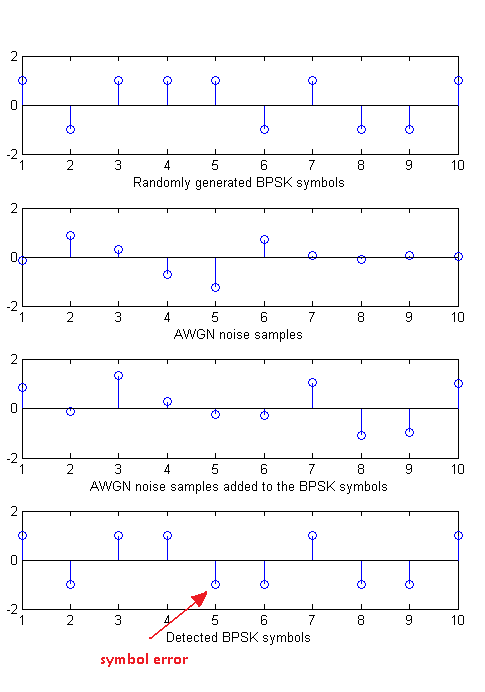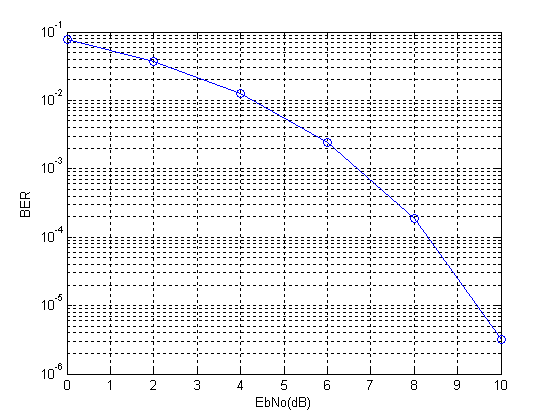Note:

1. To generate the above given bit error rate plot you would have to create a piece of code which calls the above function for each value of EbNo and stores the output BER value in an array and then plot the BER vs EbNo at the end of simulation. We leave this to  you as an exercise.

2. We have generated BPSK symbols directly instead of first generating a binary sequence. This does not matter much in this simple example but for more advanced modulation schemes we would have to first generate a binary stream and then from that the symbols.

3. We have used one sample per symbol of BPSK modulation, as shown in the figure above. But sometimes we have to select higher number of samples per symbol (usually 4 to 10) to implement some other signal processing functions.

4. Most of the concepts discussed above can be extended to other digital modulation schemes. The concepts for analog modulation schemes are somewhat different and we do not use error rates to evaluate the performance of these schemes.